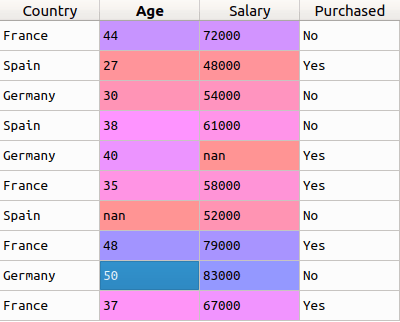You are here
Home > Data Science >

# Handle missing data in your training dataset with SciKit ImputerMost often than not, you’ll encounter a dataset  in your data science projects where you’ll have missing data in at least one column. In some cases, you can just ignore that row by taking it out of the dataset. But that’ll not be the case always. Sometimes, that row would be crucial for the training, maybe because the dataset itself is very small and you can’t afford to lose any row, or maybe it holds some important data, or for some other reason.

When this is the case, a very important question to answer is, how do you fill in the blanks? There are many approaches to solving this problem, and one of them is using SciKit’s Imputer class. If you’re interested in going through the documentation, you can find it here.

As you can see from the documentation, the constructor of the Imputer class takes in a few parameters. Let’s understand what these are:

• missing_values – This is the value which has to be replaced in the dataset. This could either be an integer, or NaN. If you don’t pass this value, NaN will be the default value. So wherever we have NaN in our dataset, the Imputer object will replace it with a new value.
• strategy – This is the strategy we’ll be using to calculate the value which has to replace the NaN occurrences in the dataset. There are three different strategies we can use:
• mean
• median
• most_frequent

“mean” is the default value here. In all three cases, the required value will be calculated and the old value will be replaced.

• axis – This can take one of two values – 0 and 1. This will decide if the Imputer will apply the strategy along the rows or along the columns. 0 for columns, and 1 for rows.
• verbose – This will just decide the verbosity of the Imputer.  By default, it’s set to 0.
• copy – This will decide if a copy of the original object has to be made, or if the Imputer should change the dataset in-place. By default, it is set to True.

Now that we know all the options given to us by the Imputer class, let’s look at an example. Consider the following dataset:As you can see from the image above, we have two ‘nan’s, one each in the second and the third columns. We need to calculate the values for these two cells. For this example, we’ll be using the strategy ‘mean’ and imputing along the columns, as imputing along the rows makes no sense here.  The code for this is as follows:

``````from sklearn.preprocessing import Imputer
imputer = Imputer(missing_values = 'NaN', strategy = 'mean', axis = 0)
imputer = imputer.fit(X[:, 1:3])
X[:, 1:3] = imputer.transform(X[:, 1:3])``````

Now, if you look at the dataset, which is stored in X, you’ll see that the two values have been replaced by the mean of all the values in their respective columns:And that’s pretty much how you could easily take care of missing data in your dataset.